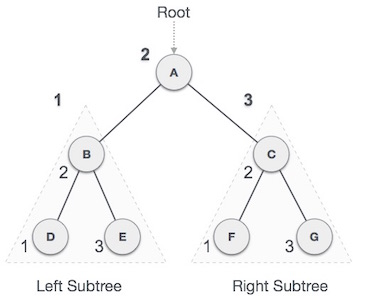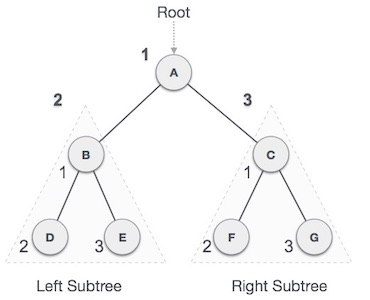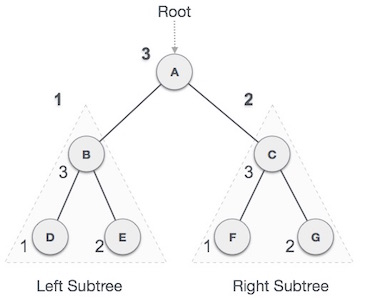### C++ Binary Tree Representation and Traversals (Code)

Binary Tree Representation and Traversals

In this article we are going to discuss the Code for Binary Tree Representation and Different types of Traversals(INORDER, PREORDER, POSTORDER).

Traversal is a process to visit all the nodes of a tree and may print their values too. Because, all nodes are connected via edges (links) we always start from the root (head) node. That is, we cannot randomly access a node in a tree. There are three ways which we use to traverse a tree

1. In-order Traversal
2. Pre-order Traversal
3. Post-order Traversal

Generally, we traverse a tree to search or locate a given item or key in the tree or to print all the values it contains.

## In-order Traversal

In this traversal method, the left subtree is visited first, then the root and later the right sub-tree. We should always remember that every node may represent a subtree itself.

If a binary tree is traversed in-order, the output will produce sorted key values in an ascending order.We start from A, and following in-order traversal, we move to its left subtree BB is also traversed in-order. The process goes on until all the nodes are visited. The output of inorder traversal of this tree will be

D->B->E->A->F->C->G

### Algorithm

`Until all nodes are traversed Step 1  Recursively traverse left subtree.Step 2  Visit root node.Step 3  Recursively traverse right subtree.`

## Pre-order Traversal

In this traversal method, the root node is visited first, then the left subtree and finally the right subtree.We start from A, and following pre-order traversal, we first visit A itself and then move to its left subtree BB is also traversed pre-order. The process goes on until all the nodes are visited. The output of pre-order traversal of this tree will be

A->B->D->E->C->F->G

### Algorithm

`Until all nodes are traversed Step 1  Visit root node.Step 2  Recursively traverse left subtree.Step 3  Recursively traverse right subtree.`

## Post-order Traversal

In this traversal method, the root node is visited last, hence the name. First we traverse the left subtree, then the right subtree and finally the root node.We start from A, and following Post-order traversal, we first visit the left subtree BB is also traversed post-order. The process goes on until all the nodes are visited. The output of post-order traversal of this tree will be

D->E->B->F->G->C->A

### Algorithm

`Until all nodes are traversed Step 1  Recursively traverse left subtree.Step 2  Recursively traverse right subtree.Step 3  Visit root node.`

CODE FOR INORDER, PREORDER, POSTORDER`/*C++ Program for different tree traversals */#include <iostream> using namespace std;/* A binary tree node has data, pointer to left child and a pointer to right child */   struct Node {     int data;     Node* left;     Node* right;      /*For initializing the Node*/    Node(int val)     {         data = val;         left = NULL;         right = NULL;     } }; /* Given a binary tree, print its nodes in preorder*/void preorder(Node* rt){	if(rt!=NULL)	{		cout<<rt->data<<" ";		preorder(rt->left);		preorder(rt->right);	}}/* Given a binary tree, print its nodes in inorder*/void inorder(Node* rt){	if(rt!=NULL)	{		inorder(rt->left);		cout<<rt->data<<" ";		inorder(rt->right);	}}/* Given a binary tree, print its nodes in postorder*/void postorder(Node* rt){	if(rt!=NULL)	{		postorder(rt->left);		postorder(rt->right);		cout<<rt->data<<" ";	}}/* DRIVER FUNCTION */int main() {    /*Insert the Elements according to the Tree (Picture)*/    Node* root = new Node(1);     root->left = new Node(2);     root->right = new Node(3);     root->left->left = new Node(4);     root->left->right = new Node(5);    cout << "\nInorder traversal of binary tree is \n";     inorder(root);     cout << "\nPreorder traversal of binary tree is \n";     preorder(root);    cout << "\npostorder traversal of binary tree is \n";     postorder(root);    return 0; } `
`Output : `
`Inorder traversal of binary tree is4 2 5 1 3Preorder traversal of binary tree is1 2 4 5 3postorder traversal of binary tree is4 5 2 3 1`#### More Articles of Shaik Ismail:

Name Views Likes
C++ Counting Sort 2027 1
C++ Shutdown Using C++ Code 5745 1
C++ Basics of Graph Data Structure 1648 0
C++ Selection Sort 1438 0
C++ Mirror Binary Tree 836 0
C++ AVL Rotations 2495 0
C++ Merge Sort 6012 0
C++ Graph Representation 703 0
C++ Insertion Sort 627 0
C++ Heap Sort 1582 0
C++ BFS in Graph 4547 0
C++ Deletion in Heaps 6538 0
C++ Check if a binary tree is a BST or not 1576 0
C++ Deletion of Nodes in BST 5297 0
C++ Identical Binary Trees 557 0
C++ Insertion of Nodes in BST 550 0
C++ Single Number LeetCode Bit Manipulation 1982 0
C++ Lowest Common Ancestor 1517 0
C++ Kth Largest Element in BST 858 0
C++ Height of the Binary Tree (Code) 976 0
C++ DFS for a Graph 6023 0
C++ Diameter of a Binary Tree 1914 0
C++ Binary Tree Representation and Traversals (Code) 665 1
C++ Basics of Tree Data Structure PART - 2 700 0
C++ Vertical Order Traversal of a Binary Tree 887 0
Basics of Tree Data Structure PART - 1 426 0
C++ Basics of Heap Data Structure 1420 0
CPP Project or program to get six subjects marks of a student and then calculate its total, average and percentage and display them on the screen 419 0
C++ Basics of BST Part - 1 750 0
C++ Searching in BST 629 0
C++ AVL Tree 883 0
Tree 447 1
C++ Missing Number(LeetCode) 2201 0
C++ Insertion of Elements in Heap 3906 0
C++ Level Order Traversal of a Binary Tree 956 0
C++ Binary Tree 722 0
C++ DNF Algorithm for Sorting 0,1,2 1780 0
C++ Wave Sort 944 0
C++ Binary Tree is a SumTree or Not 411 0
C++ Deletion in AVL Trees 686 0
C++ Quick Sort 4764 0
C++ Power of Two Leetcode BitManipulation 1000 0
C++ Bottom View of a Binary Tree 437 0
C++ Kth Smallest Element in BST 960 0
C++ Bubble Sort 713 0
C++ Top View of a Binary Tree 488 0
C++ Majority Element (LeetCode) 1043 0
C++ Sum of all leaf nodes 414 0
C++ Graph Representation (Adjacency list) 2736 0
C++ Count set bits in an integer 1510 0